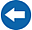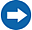Home >Tips >SharePoint Flows >Calculate sums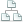Site map

# Calculate Sums and Show Totals

## A SharePoint Flows tutorial by Peter Kalmström

This article solves a problem with calculated columns: they cannot be combined with the Totals view.

The Total Cost column in the image below calculates its values from the Hardware and Setup costs of the same item. Therefore the sum of all total costs cannot be displayed on top, as for the Hardware and Setup costs. When you use a column with a calculated value in a SharePoint list, you cannot combine it with the Totals view.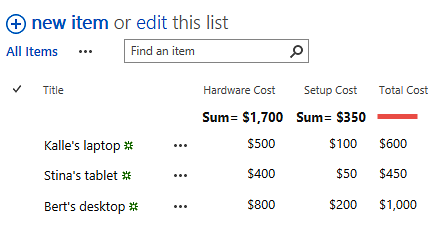The Totals view displays a calculated value (the average, count, maximum, minimum, sum, standard deviation or variance) for each column with numbers or currency above these columns, so it is very useful. You would really want to have Totals for a calculated column that contains numbers also. Solve the problem by letting a flow do the calculation and update the column. Then you don't have to use a calculated column at all!

Unfortunately the Totals view can only be used in lists with the classic interface when this is written, but it will hopefully soon be added to modern lists too.In the demo below, Peter Kalmström, CEO and Systems Designer of kalmstrom.com Business Solutions, shows how to create a flow for calculation. The flow calculates the sum of values in two currency columns in a newly created or modified item a SharePoint list and updates a third currency column. This way you can have a "row sum" in SharePoint without using a calculated column.

As Peter is using a currency column for the result of he calculation, and not a calculated column, he can use the Totals view without problems and see the Totals for the flow calculated column.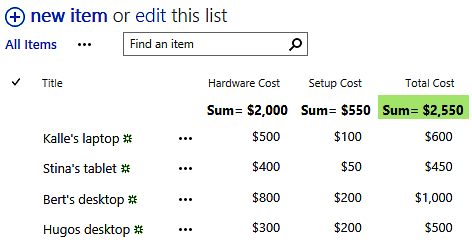### Steps to create a flow that calculates sums from column values

1. Create a Number or Currency column for the list you want to use.
2. Create a flow from blank and select the trigger 'SharePoint, When an item is created or modified'.
3. When you have entered SharePoint site and list name, add the action 'SharePoint - Update item' for the same site and list.
4. Add the dynamic contents 'ID' and 'Title'.
5. Create an expression for the field that should be calculated: add + the dynamic contents for the columns that you want to include in the calculation. Separate them with comma.
6. Save and test the flow.